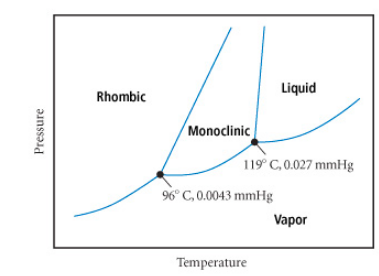# Problem: The phase diagram for sulfur is shown below. The rhombic and monoclinic phases are two solid phases.Below what pressure will solid sulfur sublime?Express your answer using two significant figures.

###### FREE Expert Solution

Sublimation

• process of going from solid to gas###### Problem Details

The phase diagram for sulfur is shown below. The rhombic and monoclinic phases are two solid phases.

Below what pressure will solid sulfur sublime?What scientific concept do you need to know in order to solve this problem?

Our tutors have indicated that to solve this problem you will need to apply the Phase Diagram concept. You can view video lessons to learn Phase Diagram. Or if you need more Phase Diagram practice, you can also practice Phase Diagram practice problems.

What is the difficulty of this problem?

Our tutors rated the difficulty ofThe phase diagram for sulfur is shown below. The rhombic and...as medium difficulty.

How long does this problem take to solve?

Our expert Chemistry tutor, Sabrina took 2 minutes and 22 seconds to solve this problem. You can follow their steps in the video explanation above.

What professor is this problem relevant for?

Based on our data, we think this problem is relevant for Professor Logan's class at SUNY College at Brockport.